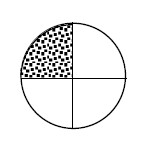### Home > PC > Chapter 3 > Lesson 3.1.2 > Problem3-25

3-25.

A circle has a radius of $10$ cm.1. Find the circumference and area of the circle using exact values.

$\text{circumference} = \left(2\right)\left(π\right)\left(\text{radius}\right)$

$\text{area} = π\left(\text{radius}\right)^{2}$

2. Find the area and arc length of the non-shaded portion.

What part of the circumference and area is needed?